# Data Exploration with Python

In this post, we will explore a dataset using Python. The dataset we will use is the Ghouls, Goblins, and Ghost (GGG) dataset available at the kaggle website. The analysis will not be anything complex we will simply do the following.

• Data preparation
• Data  visualization
• Descriptive statistics
• Regression analysis

Data PreparationThe GGG dataset is fictitious data on the characteristics of spirits. Below are the modules we will use for our analysis.

```import pandas as pd
import statsmodels.regression.linear_model as sm
import numpy as np```

`df=pd.read_csv('FILE LOCATION HERE')`

We store the data as “df” in the example above. Next, we will take a peek at the first few rows of data to see what we are working with.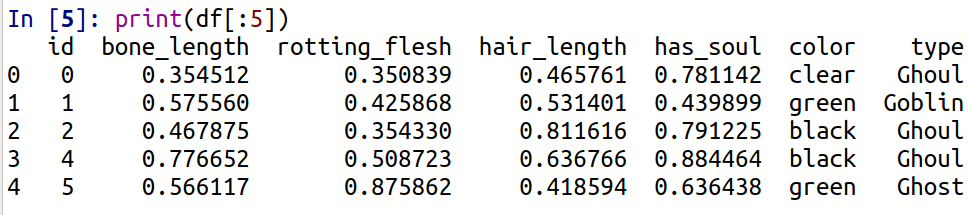Using the print function and accessing the first five rows reveals. It appears the first five columns are continuous data and the last two columns are categorical. The ‘id’ variable is useless for our purposes so we will remove it with the code below.

`df=df.drop(['id'],axis=1)`

The code above uses the drop function to remove the variable ‘id’. This is all saved into the object ‘df’. In other words, we wrote over are original ‘df’.

Data Visualization

We will start with our categorical variables for the data visualization. Below is a table and a graph of the ‘color’ and ‘type’ variables.

First, we make an object called ‘spirits’ using the groupby function to organize the table by the ‘type’ variable.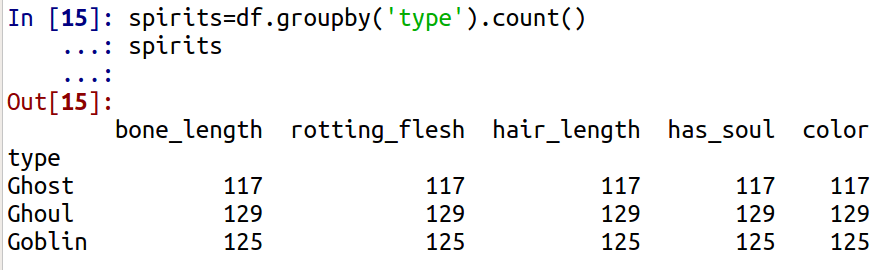Below we make a graph of the data above using the .plot function. A professional wouldn’t make this plot but we are just practicing how to code.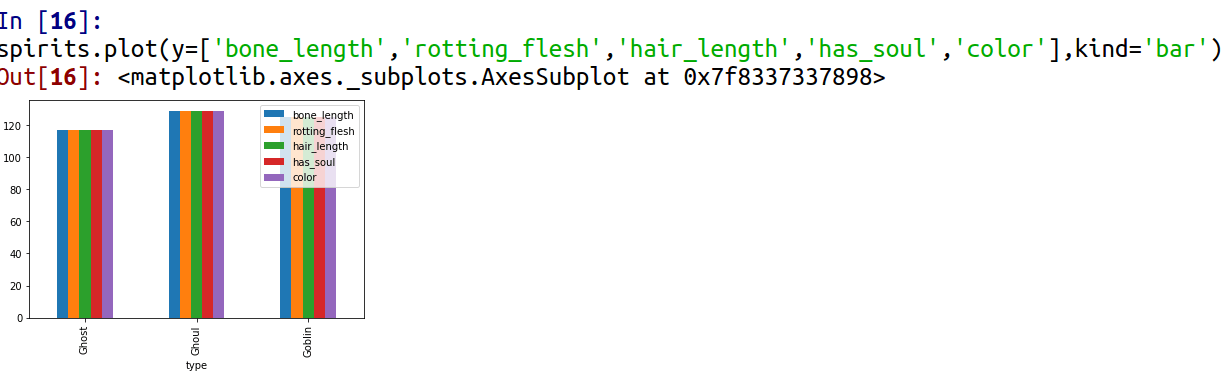We now know how many ghosts, goblins and, ghouls there are in the dataset. We will now do a breakdown of ‘type’ by ‘color’ using the .crosstab function from pandas.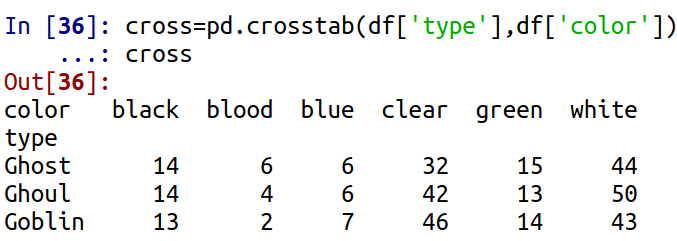We will now make bar graphs of both of the categorical variables using the .plot function.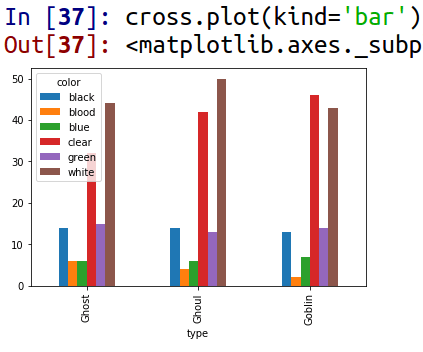We will now turn our attention to the continuous variables. We will simply make histograms and calculate the correlation between them. First the histograms

The code is simply subset the variable you want in the brackets and then type .plot.hist() to access the histogram function. It appears that all of our data is normally distributed. Now for the correlation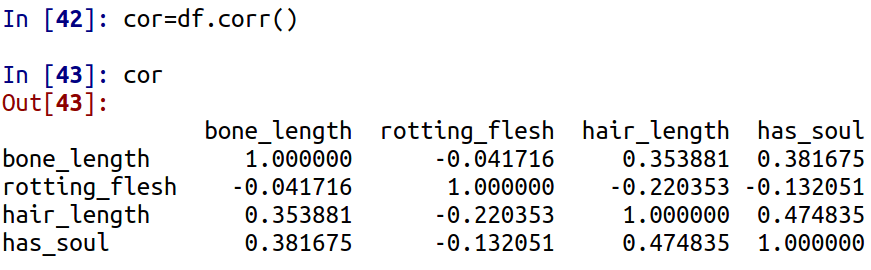Using the .corr() function has shown that there are now high correlations among the continuous variables. We will now do an analysis in which we combine the continuous and categorical variables through making boxplots

The code is redundant. We use the .boxplot() function and tell python the column which is continuous and the ‘by’ which is the categorical variable.

Descriptive Stats

We are simply going to calcualte the mean and standard deviation of the continuous variables.

```df["bone_length"].mean()
Out: 0.43415996604821117

np.std(df["bone_length"])
Out: 0.13265391313941383

df["hair_length"].mean()
Out: 0.5291143100058727

np.std(df["hair_length"])
Out: 0.16967268504935665

df["has_soul"].mean()
Out: 0.47139203219259107

np.std(df["has_soul"])
Out: 0.17589180837106724```

The mean is calcualted with the .mean(). Standard deviation is calculated using the .std() function from the numpy package.

Multiple Regression

Our final trick is we want to explain the variable “has_soul” using the other continuous variables that are available. Below is the code

```X = df[["bone_length", "rotting_flesh","hair_length"]]

y = df["has_soul"]

model = sm.OLS(y, X).fit()```

In the code above we crate to new list. X contains are independent variables and y contains the dependent variable. Then we create an object called model and use the  OLS() function. We place the y and X inside the parenthesis and we then use the .fit() function as well. Below is the summary of the analysis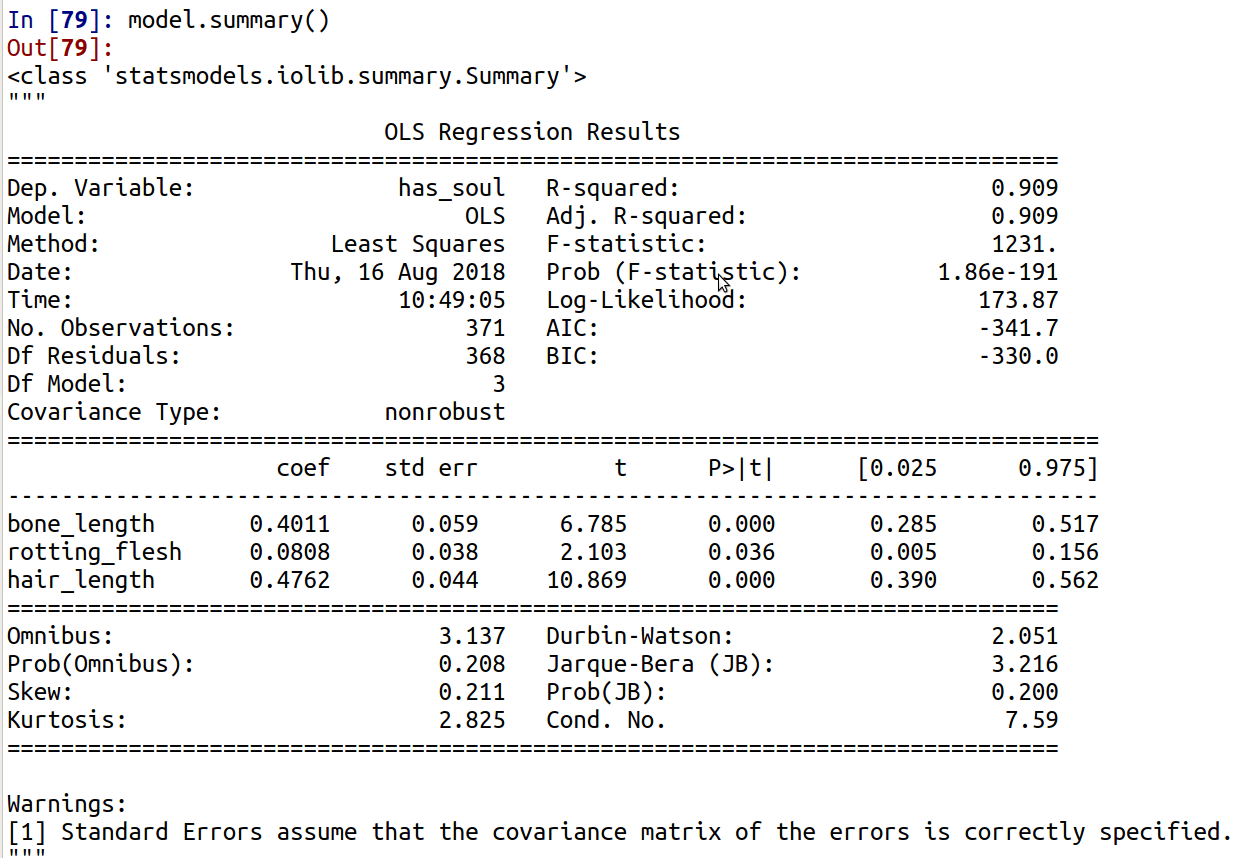There is obviously a lot of information in the output. The r-square is 0.91 which is surprisingly high given that there were not high correlations in the matrix. The coefficiencies for the three independent variables are listed and all are significant. The AIC and BIC are for model comparison and do not mean much in isolation. The JB stat indicates that are distribution is not normal. Durbin watson test indicates negative autocorrelation which is important in time-series analysis.

Conclusion

Data exploration can be an insightful experience. Using Python, we found mant different patterns and ways to describe the data.